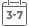# Probability, Statistics, and Stochastic Processes

• Publish Date: 2005-06-14
• Binding: Hardcover
• Author: Peter Olofsson
• Sale
• \$29.81
• Regular price \$184.46A mathematical and intuitive approach to probability, statistics, and stochastic processes

This textbook provides a unique, balanced approach to probability, statistics, and stochastic processes. Readers gain a solid foundation in all three fields that serves as a stepping stone to more advanced investigations into each area. This text combines a rigorous, calculus-based development of theory with a more intuitive approach that appeals to readers' sense of reason and logic, an approach developed through the author's many years of classroom experience.

The text begins with three chapters that develop probability theory and introduce the axioms of probability, random variables, and joint distributions. The next two chapters introduce limit theorems and simulation. Also included is a chapter on statistical inference with a section on Bayesian statistics, which is an important, though often neglected, topic for undergraduate-level texts. Markov chains in discrete and continuous time are also discussed within the book.

More than 400 examples are interspersed throughout the text to help illustrate concepts and theory and to assist the reader in developing an intuitive sense of the subject. Readers will find many of the examples to be both entertaining and thought provoking. This is also true for the carefully selected problems that appear at the end of each chapter.

This book is an excellent text for upper-level undergraduate courses. While many texts treat probability theory and statistical inference or probability theory and stochastic processes, this text enables students to become proficient in all three of these essential topics. For students in science and engineering who may take only one course in probability theory, mastering all three areas will better prepare them to collect, analyze, and characterize data in their chosen fields.

MORE FROM THIS COLLECTION